MATLAB Function Referenceatan2

Four-quadrant inverse tangent

Syntax

• ```P = atan2(Y,X)
```

Description

```P = atan2(Y,X) ``` returns an array `P` the same size as `X` and `Y` containing the element-by-element, four-quadrant inverse tangent (arctangent) of the real parts of `Y` and `X.` The elements of `P` are in the interval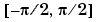. Any imaginary parts of the inputs are ignored.

Elements of `P` lie in the closed interval `[-pi,pi]`, where `pi` is the MATLAB floating-point representation of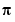. `atan` uses `sign(Y)` and `sign(X)` to determine the specific quadrant.

```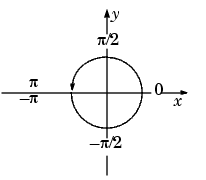```

`atan2(Y,X)` contrasts with `atan(Y/X)`, whose results are limited to the interval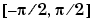, or the right side of this diagram.

Examples

Any complex number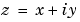is converted to polar coordinates with

• ```r = abs(z)
theta = atan2(imag(z)`,`real(z))
```

For example,

• ```z = 4 + 3i;
r = abs(z)
theta = atan2(imag(z)`,`real(z))

r =
5

theta =
0.6435
```

This is a common operation, so MATLAB provides a function, `angle(z)`, that computes `theta = atan2(imag(z),real(z))`.

To convert back to the original complex number

• ```z = r *exp(i *theta)
z =

4.0000 + 3.0000i
```

Algorithm

`atan2` uses FDLIBM, which was developed at SunSoft, a Sun Microsystems, Inc. business, by Kwok C. Ng, and others. For information about FDLIBM, see http://www.netlib.org.

See Also

`angle`, `atan`, `atanh`

© 1994-2005 The MathWorks, Inc.